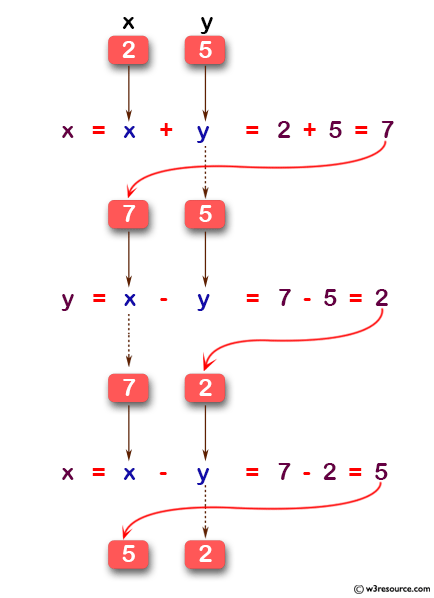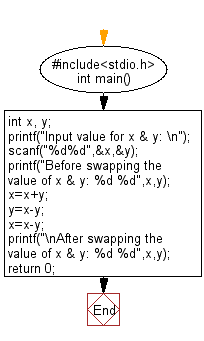﻿ C Program: Swaps two numbers without using third variable - w3resource

# C Exercises: Swaps two numbers without using third variable

## C Basic Declarations and Expressions: Exercise-55 with Solution

Write a C program that swaps two numbers without using a third variable.

Pictorial Presentation:Sample Solution:

C Code:

``````#include<stdio.h>
int main() {
int x, y;

// Prompt user to input values for x and y
printf("Input value for x & y: \n");
scanf("%d%d",&x,&y);

// Display the values of x and y before swapping
printf("Before swapping the value of x & y: %d %d",x,y);

// Swap the values of x and y using arithmetic operations
x = x + y;
y = x - y;
x = x - y;

// Display the values of x and y after swapping
printf("\nAfter swapping the value of x & y: %d %d",x,y);

return 0;
}
``````

Sample Output:

```Input value for x & y:
Before swapping the value of x & y: 5 7
After swapping the value of x & y: 7 5
```

Flowchart:C programming Code Editor:

What is the difficulty level of this exercise?

Test your Programming skills with w3resource's quiz.

﻿